## 2.3 Creating a Bar Graph

### 2.3.1 Problem

You want to make a bar graph.

### 2.3.2 Solution

To make a bar graph of values (Figure 2.5, left), use `barplot()` and pass it a vector of values for the height of each bar and (optionally) a vector of labels for each bar. If the vector has names for the elements, the names will automatically be used as labels:

``````# First, take a look at the BOD data
BOD
#>   Time demand
#> 1    1    8.3
#> 2    2   10.3
#> 3    3   19.0
#> 4    4   16.0
#> 5    5   15.6
#> 6    7   19.8``````
``barplot(BOD\$demand, names.arg = BOD\$Time)``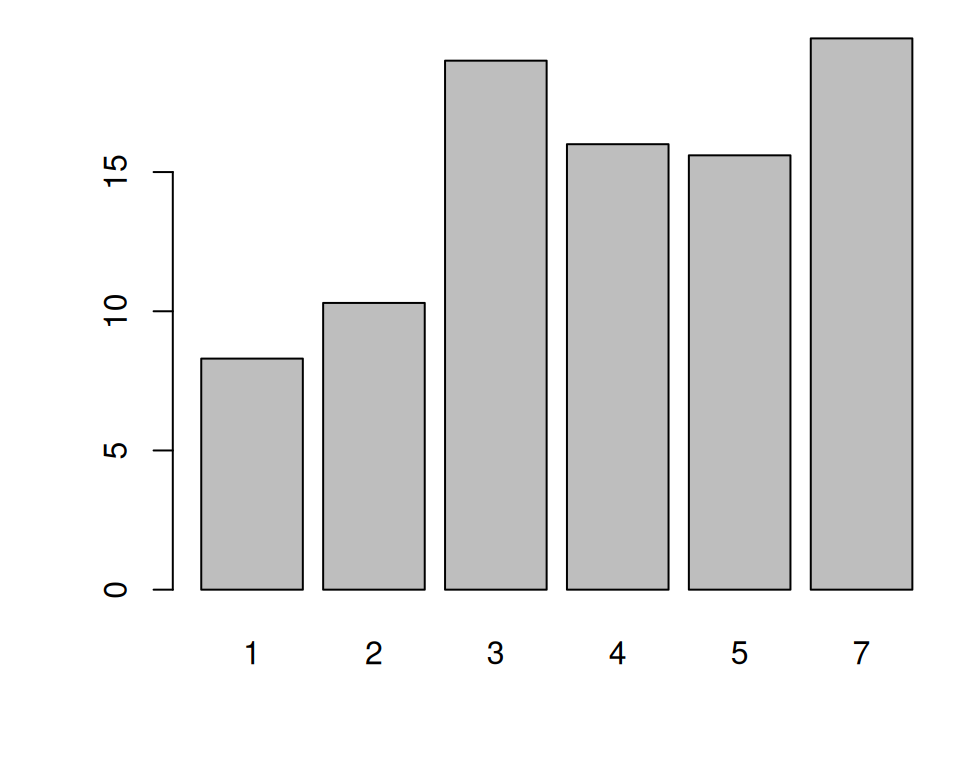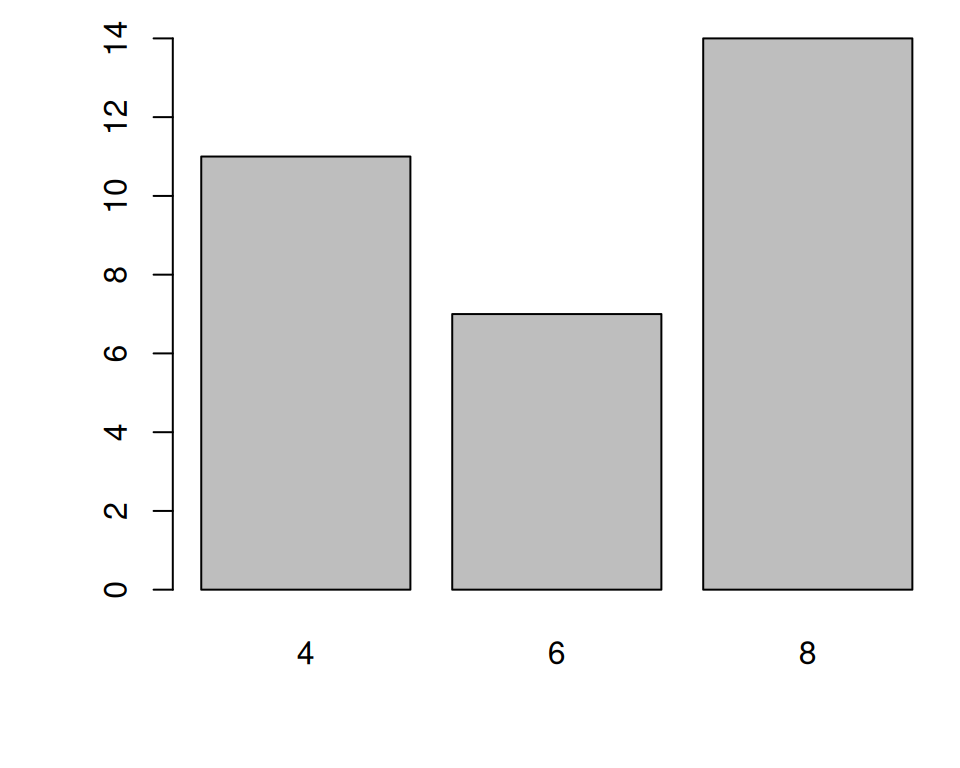Figure 2.5: Bar graph of values with base graphics (left); Bar graph of counts (right)

Sometimes “bar graph” refers to a graph where the bars represent the count of cases in each category. This is similar to a histogram, but with a discrete instead of continuous x-axis. To generate the count of each unique value in a vector, use the `table()` function:

``````# There are 11 cases of the value 4, 7 cases of 6, and 14 cases of 8
table(mtcars\$cyl)
#>
#>  4  6  8
#> 11  7 14``````

Then pass the table to `barplot()` to generate the graph of counts:

``````# Generate a table of counts
barplot(table(mtcars\$cyl))``````

With ggplot2, you can get a similar result using `geom_col()` (Figure 2.6). To plot a bar graph of values, use `geom_col()`. Notice the difference in the output when the x variable is continuous and when it is discrete:

``````library(ggplot2)

# Bar graph of values. This uses the BOD data frame, with the
# "Time" column for x values and the "demand" column for y values.
ggplot(BOD, aes(x = Time, y = demand)) +
geom_col()

# Convert the x variable to a factor, so that it is treated as discrete
ggplot(BOD, aes(x = factor(Time), y = demand)) +
geom_col()``````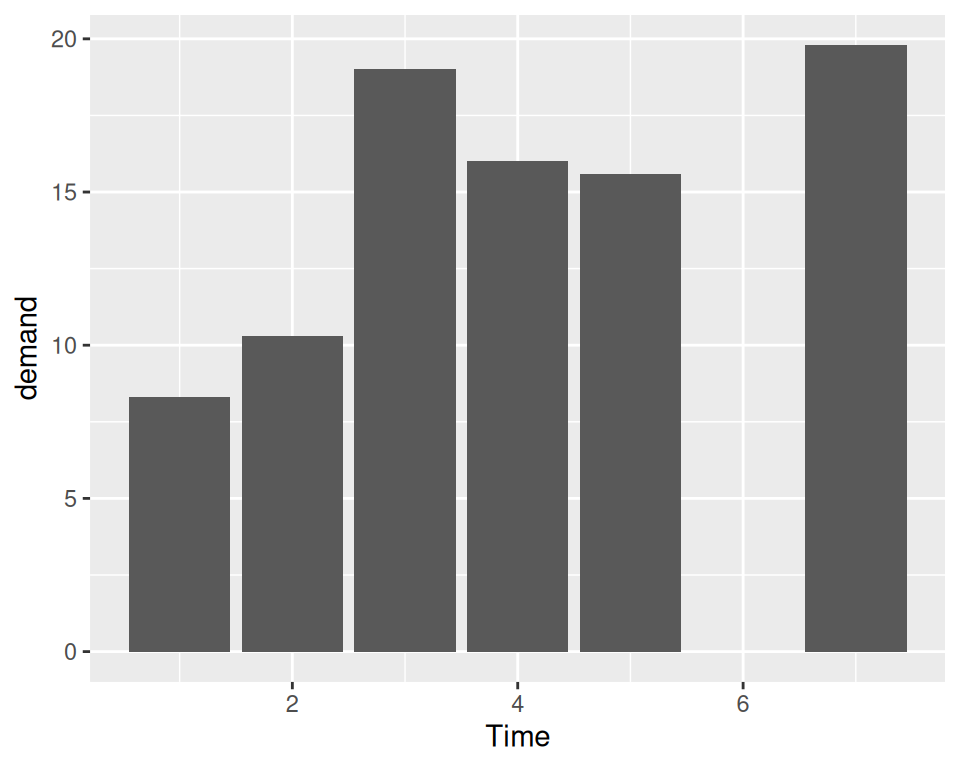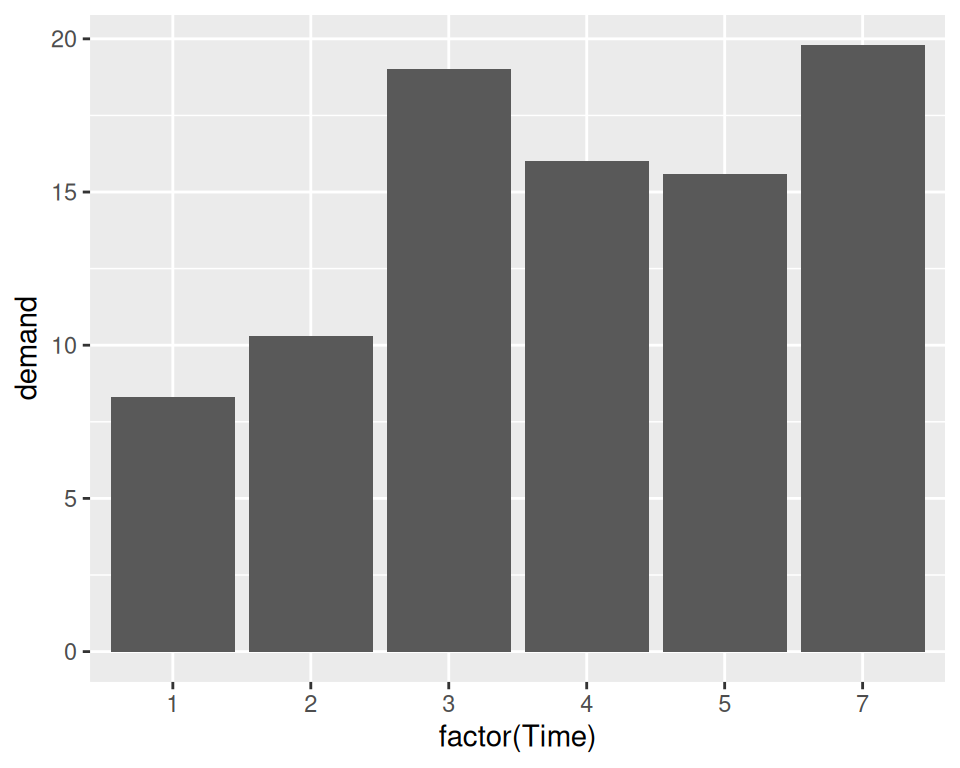Figure 2.6: Bar graph of values using `geom_col()` with a continuous x variable (left); With x variable converted to a factor (notice that there is no entry for 6; right)

ggplot2 can also be used to plot the count of the number of data rows in each category (Figure 2.7, by using `geom_bar()` instead of `geom_col()`. Once again, notice the difference between a continuous x-axis and a discrete one. For some kinds of data, it may make more sense to convert the continuous x variable to a discrete one, with the `factor()` function.

``````# Bar graph of counts This uses the mtcars data frame, with the "cyl" column for
# x position. The y position is calculated by counting the number of rows for
# each value of cyl.
ggplot(mtcars, aes(x = cyl)) +
geom_bar()

# Bar graph of counts
ggplot(mtcars, aes(x = factor(cyl))) +
geom_bar()``````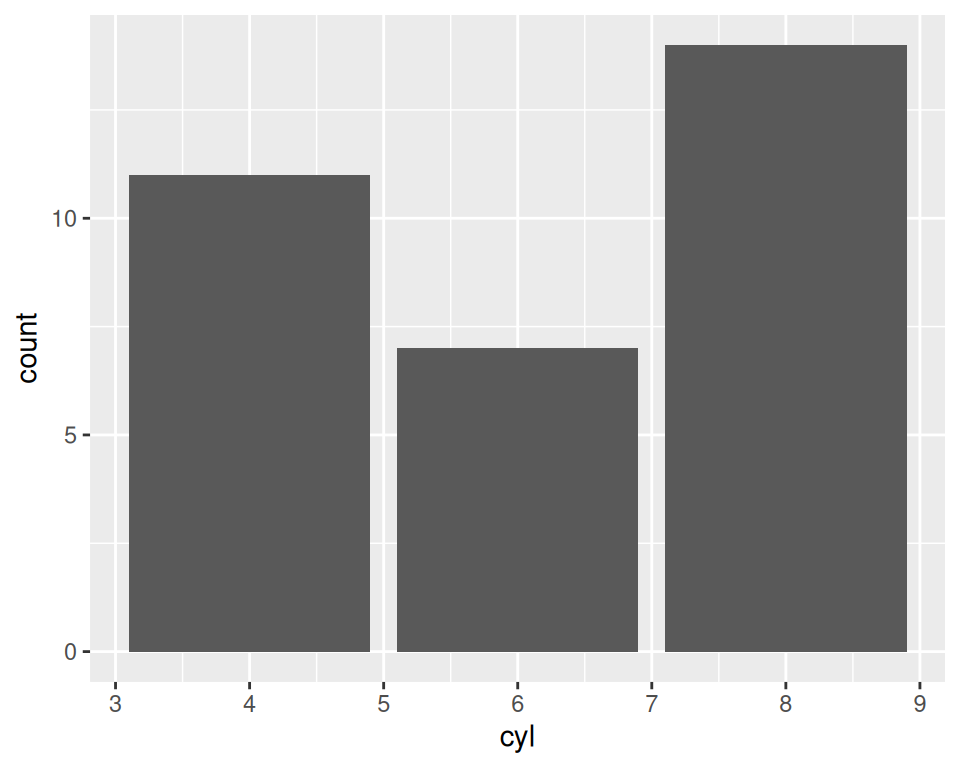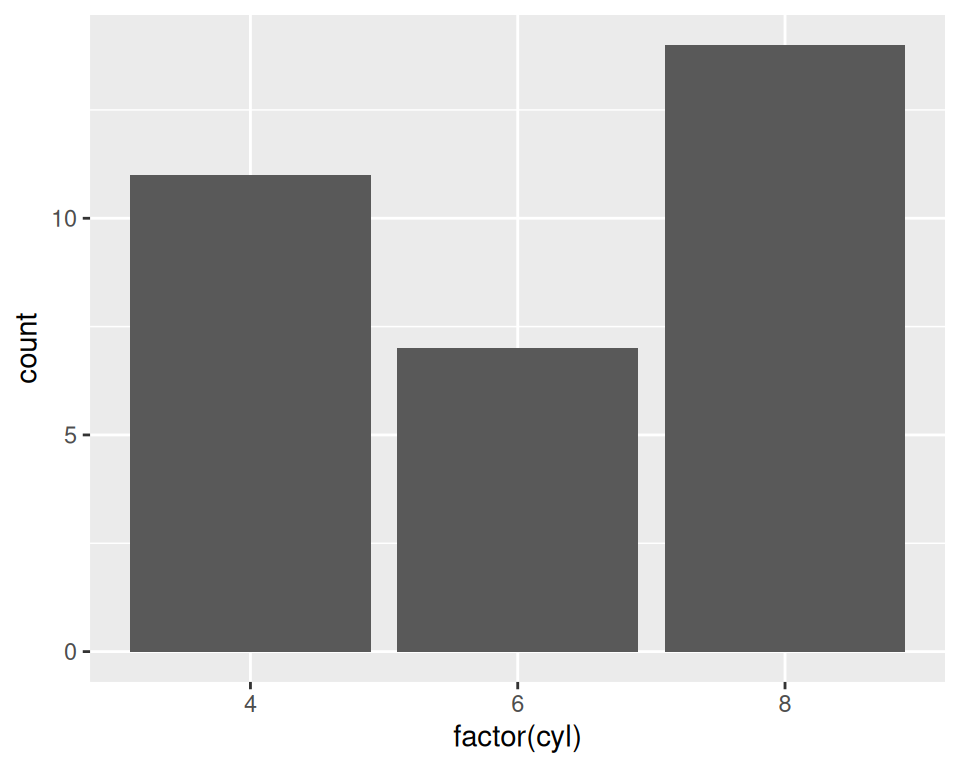Figure 2.7: Bar graph of counts using `geom_bar()` with a continuous x variable (left); With x variable converted to a factor (right)

Note

In previous versions of ggplot2, the recommended way to create a bar graph of values was to use `geom_bar(stat = "identity")`. As of ggplot2 2.2.0, there is a `geom_col()` function which does the same thing.